#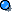Single object in motion Given mass and momentum, solve for velocity p=mv v=p/m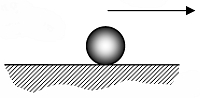Inelastic  collision (sticks) – one object stationary Given initial velocity and masses, solve for final momentum  pinitial = m1v1initial + m2v2initial pinitial = m1v1initial + 0 = pfinal pfinal = m1v1initialGiven initial velocity and masses, solve for final velocity m1v1initial + m2v2initial = (m1+m2)v(1+2)final v(1+2)final = (m1v1initial) / (m1+m2)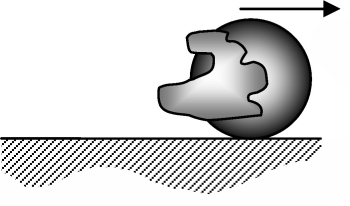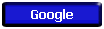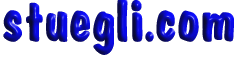Copyright © 2005 -  S. B. EglI